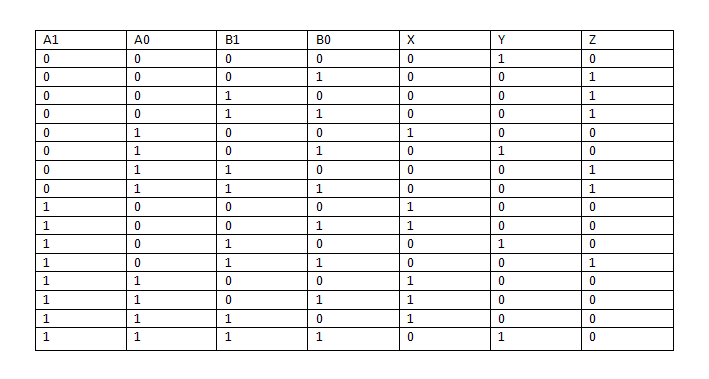# Comparator of 2 bit numbers

< Mentor Note -- thread moved to HH from the technical engineering forums, so no HH Template is shown >

Is there a way to create two bit numbers comparator using one 2 to 4 decoder and standard logic gates?

Last edited by a moderator:

mfb
Mentor
I would avoid the decoder because it is easier without, but it is possible in both cases (and the decoder just has standard logic gates anyway).

analogdesign
used a K-map to figure out how to do it with logic gates. The decoder will only be useful in the sense it can be used to implement certain logic equations.

berkeman
Mentor
Is there a way to create two bit numbers comparator using one 2 to 4 decoder and standard logic gates?

Do you mean a logic block that takes in two 2-bit numbers, and has one output that is either high or low depending on the relative magnitude of the two 2-bit numbers? What if they are equal? Can you show us a truth table for the function that you want to implement?

And is this for a synchronous (clocked) application, or does it need to run asynchronously?

Baluncore
2021 Award
Is there a way to create two bit numbers comparator using one 2 to 4 decoder and standard logic gates?
Yes there is. But it is easier to do it with a 1 of 16 data selector and no gates than with a data decoder.

But two XOR gates driving one NOR gate would achieve the same.

Do you mean a logic block that takes in two 2-bit numbers, and has one output that is either high or low depending on the relative magnitude of the two 2-bit numbers? What if they are equal? Can you show us a truth table for the function that you want to implement?

And is this for a synchronous (clocked) application, or does it need to run asynchronously?This is the truth table. X is active if the A1A0 is greater that B1B0 if they have the same value Y is active and if B1B0 is greater than A1A0 then Z is active. Problem is, that i have to use 2 to 4 decoder and i don't know how to make only two inputs since i have 4(A1, A0, B1 and B0) and make it work this way.

mfb
Mentor
Connect the decoder to something and ignore its output, if the decoder has to appear in the system?
Well, you can connect it to some part of the input, and use its internal gates (C1 AND C0, C1 AND NOT C0, ...) as intermediate results.

It is possible to produce all outputs with 12 logic gates, not including NOT, without the decoder. Fewer if you count XOR as one, or use gates with more than 2 inputs.

Connect the decoder to something and ignore its output, if the decoder has to appear in the system?
Well, you can connect it to some part of the input, and use its internal gates (C1 AND C0, C1 AND NOT C0, ...) as intermediate results.

It is possible to produce all outputs with 12 logic gates, not including NOT, without the decoder. Fewer if you count XOR as one, or use gates with more than 2 inputs.

Decoder has to appear in system, and it has to be 2 to 4 decoder and standard logical gates are allowed but still i don't understand how can i do it with just one decoder and logic gates.

berkeman
Mentor
Well, just use the single decoder with the first 2 inputs, and then write a truth table using the 4 outputs of that decoder and the other 2 inputs. Kind of a dorky way to have to do it, but it can work.

Alternately, use the decoder to generate the outputs, and use logic gates on the 4 inputs to generate the 2-bit input to the decoder.

Try writing the new truth tables for each of those options, to see which uses fewer gates (probably the second option will be better, but that's just a guess).

mfb
Mentor
Decoder has to appear in system, and it has to be 2 to 4 decoder and standard logical gates are allowed but still i don't understand how can i do it with just one decoder and logic gates.
You can reproduce every truth table with logic gates. This can be done in more or less efficient ways, but it is always possible and there is a direct and natural way to find a solution. And as mentioned, the decoder can be used somewhere in the process.

Well, just use the single decoder with the first 2 inputs, and then write a truth table using the 4 outputs of that decoder and the other 2 inputs. Kind of a dorky way to have to do it, but it can work.

Alternately, use the decoder to generate the outputs, and use logic gates on the 4 inputs to generate the 2-bit input to the decoder.

Try writing the new truth tables for each of those options, to see which uses fewer gates (probably the second option will be better, but that's just a guess).

Thanks, i will try to do it that way.